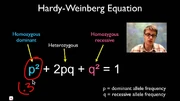## FANDOM

419 PagesHardy-Wineberg Principle

Write the first paragraph of your page here.

## What is a lethal mutation?Edit

They are one of those random mutations or changes of alleles during meiosis. Mutations passed on to the next generation are considered evolution, but not all evolution is good. In the case of lethal mutations it is a mutation that is detrimental to the species and eventually leads to extinction in the organisms that possess that particular mutation.

## Dominant Lethal GenesEditEdit

Dominant lethal genes are expressed in both homozygotes and heterozygotes. But how can alleles like this be passed from one generation to the next if they cause death? Dominant lethal genes are rarely detected due to their rapid elimination from populations. One example of a disease caused by a dominant lethal allele is Huntington's disease, a neurological disorder in humans, which reduces life expectancy. Because the onset of Huntington's disease is slow, individuals carrying the allele can pass it on to their offspring. This allows the allele to be maintained in the population. Dominant traits can also be maintained in the population through recurrent mutations or if the penetrance of the gene is less than 100%.

Recessive Lethal Genes

Cuénot and Baur discovered recessive lethal genes in the early 1900's as they altered Mendelian inheritance ratios. Recessive lethal genes can code for either dominant or recessive traits, but they do not actually cause death unless an organism carries two copies of the lethal allele. Examples of human diseases caused by recessive lethal alleles include cystic fibrosis, sickle-cell anemia, and achondroplasia. Achondroplasia is an autosomal dominant bone disorder that causes dwarfism. While the inheritance of one achondroplasia allele can cause the disease, the inheritance of two recessive lethal alleles is fatal.

### The Hardy-Wineberg PrincipleEdit

The Hardy-Wineberg Principle can help us calculate mutations in a population of invidivduals. They showed that most copies of harmful recessive alleles are found in heterozygotes and are thus protected from the effects
of natural selection.

The Hardy-Weinberg equation is a mathematical equation that can be used to calculate the genetic variation of a population at equilibrium. In 1908, G. H. Hardy and Wilhelm Weinberg independently described a basic principle of population genetics, which is now named the Hardy-Weinberg equation. The equation is an expression of the principle known as Hardy-Weinberg equilibrium, which states that the amount of genetic variation in a population will remain constant from one generation to the next in the absence of disturbing factors.

To explore the Hardy-Weinberg equation, we can examine a simple genetic locus at which there are two alleles, A and a. The Hardy-Weinberg equation is expressed as:

p2 + 2pq + q2 = 1

where p is the frequency of the "A" allele and q is the frequency of the "a" allele in the population. In the equation, p2 represents the frequency of the homozygous genotype AA, q2 represents the frequency of the homozygous genotype aa, and 2pq represents the frequency of the heterozygous genotype Aa. In addition, the sum of the allele frequencies for all the alleles at the locus must be 1, so p + q = 1. If the p and q allele frequencies are known, then the frequencies of the three genotypes may be calculated using the Hardy-Weinberg equation. In population genetics studies, the Hardy-Weinberg equation can be used to measure whether the observed genotype frequencies in a population differ from the frequencies predicted by the equation. (http://www.nature.com/scitable/definition/hardy-weinberg-equation-299)

http://www.youtube.com/watch?v=xPkOAnK20kw#    (a great video on how to use the Hardy-Weinberg formula)

OR